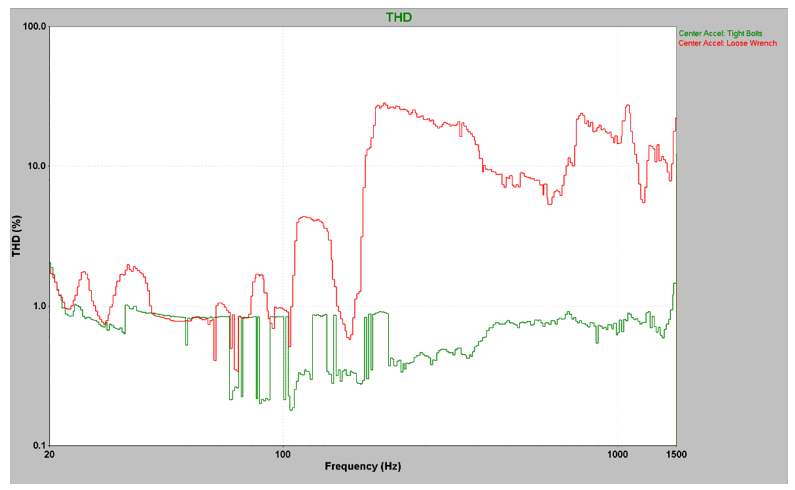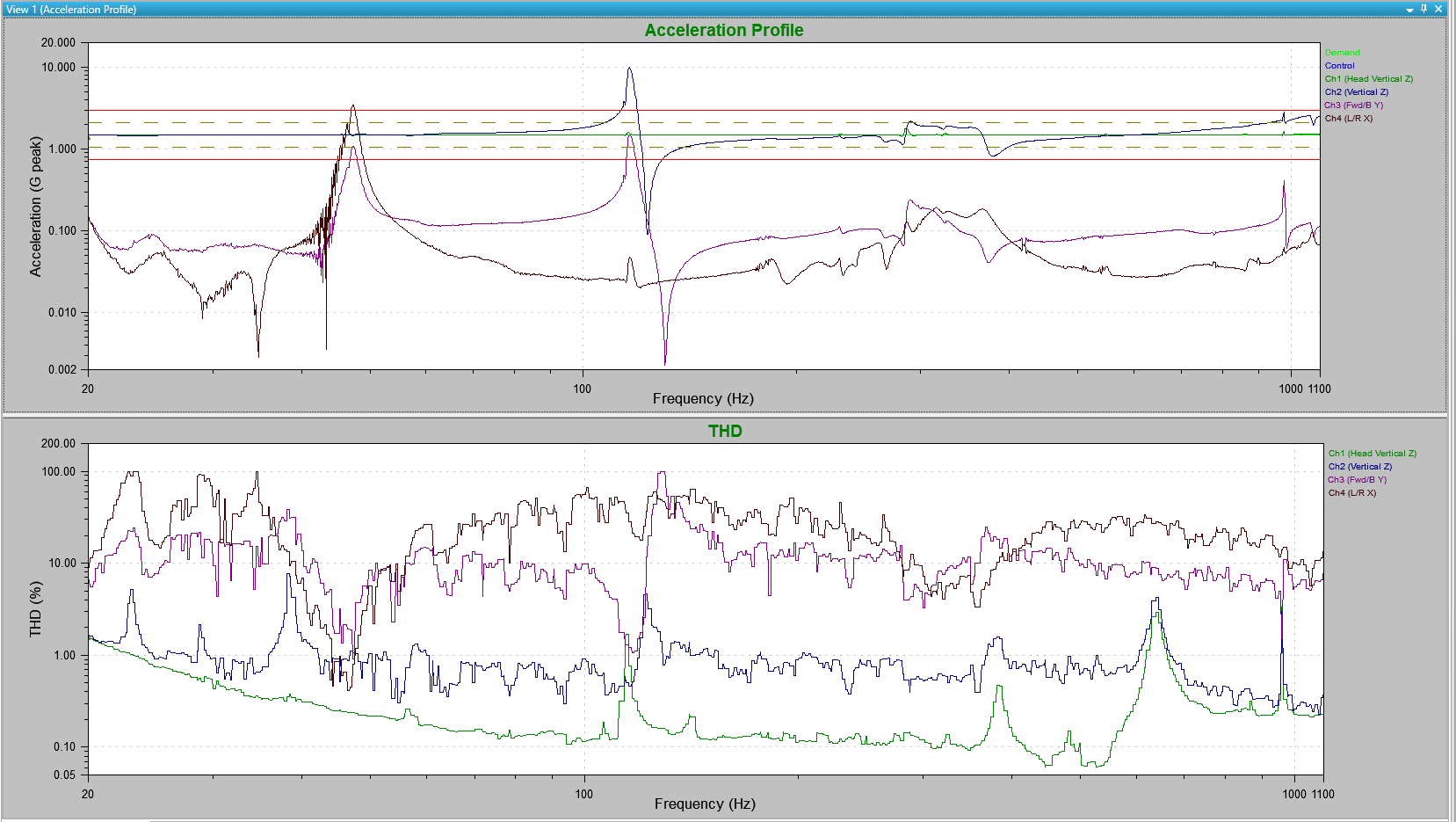# Total Harmonic Distortion

January 3, 2019

The total harmonic distortion (THD) plot is available in the sine test module when the Analyzer key is turned on. THD measures how accurately a vibration system reproduces the output signal from a controller.

In VibrationVIEW, THD is defined as the ratio of the RMS acceleration of the first ten harmonic frequencies (starting at the second harmonic) over the RMS acceleration of the fundamental frequency.

For example, if you have a 60Hz sine tone, the harmonics of 60Hz would be whole number multiples of the fundamental, such as 120Hz (60 x 2), 180Hz (60 x 3), 240Hz (60 x 4), etc. Any energy in the harmonics will result in a distorted sine wave signal.

The THD, then, is a measure of harmonic content in a signal.

In an ideal world, a pure sine tone is perfectly smooth. In the real world, however, a number of events affect the quality of the sine tone and cause distortion. The distortion is due to any harmonic content in the signal. Distortion can come from the system, such as the amplifier shaker, the device under test (DUT), or the accelerometer.

A large THD value (>10%) indicates there is significant harmonic content in the sine signal, possibly due to some weakness or malfunction in the shaker system. Engineers should expect a high THD value if two unrelated signals, such as two axes, are compared.

### THD for Analysis

THD can be used to measure how accurately a vibration system is reproducing the output signal from a controller. A small THD value indicates that the system is performing correctly, whereas a large THD value is indicative of a problem with the system. THD values can help a test engineer identify the presence of noise or unwanted vibrations in the signal. Problems in the system such as these will result in inaccurate and invalid test results.

When an engineer compares two signals that should have similar vibration, a low THD value is to be expected. Potential uses for THD are shaker validation testing, fixture mapping, and product screening.

When two signals that should have different vibrations are compared, a high THD is expected. THD may be used to observe high values in multi-axis testing. For example, a shaker vibrating with a pure sine tone in the vertical axis should excite a product in the vertical axis. However, an accelerometer measuring the vibration in the horizontal axis should not pick up much horizontal vibration, which would produce a high THD value.

### THD for Validation

To demonstrate how THD can be helpful as a tool for shaker validation testing, consider the following example.

Two tests were run on a shaker: one with a shaker in a healthy state and the second with a loose piece of equipment rattling on the head expander (similar to a loose bolt or a damaged fixture/shaker). When the THD of the head expander with all bolts firmly tightened (green) is compared to the THD of the same setup with a loose wrench on the head expander (red), it is clear that the loose wrench produces a much different response than the healthy shaker (Figure 2.8).Figure 2.8. THD vs. Frequency for two tests. The green line represents a healthy shaker system and the red line represents a damaged shaker/fixture, a loose bolt, etc.

To demonstrate how THD can be helpful as a tool in multi-axis testing, consider the following example.

An aluminum mass was mounted on a thin steel rod vibrating in the horizontal plane (Figure 2.9). A triaxial accelerometer was mounted on the end of the horizontal mass.

The THD values in the three axes at the end of the mass were compared to the vibrations of the shaker head (Figure 2.10). As shown in the graph, the vertical axis vibrations that were moving in the same plane as the shaker head produced a THD similar to the shaker head across the spectrum. The graph also shows that the other two axes produced different THD values than the shaker head across the spectrum. This indicates that there was no significant vibration in those planes at those frequencies.

The other axes had similar THD values as the shaker head at only two frequencies (approximately 45Hz and 110Hz). This indicates that the energy of vibrations in the vertical plane excited vibrations in the other planes at those frequencies.Figure 2.10. THD of three axes at the end of a large horizontal mass atop of thin steel shaft vibrating in the vertical plane. The vertical axis of the end of the mass has a similar THD as the vertical vibrating shaft. The other two axes have much higher THD values.

### Conclusion

THD can be used to compare how accurately a vibration system is reproducing the output signal from a controller. Therefore, it is useful for evaluating the quality of a controller, determining the health of a shaker system, and locating unwanted vibrations in a DUT. For example, one can use THD to compare how a shaker system is functioning from year to year. A simple way to perform a shaker validation test is to run a sine sweep across the frequency spectrum of the shaker and evaluate the THD of the system. Once a base-line THD is established, future THD responses can be compared to the original base-line value to evaluate the shaker system’s health.

THD can also be used in multi-axis situations to determine if vibrations in one axis are affecting the vibrations in another axis. It provides a quick, visual reference that identifies any frequencies of interest.